# AlgebraPage 7

#### WATCH ALL SLIDES

Example 6-1a

Method 1 Write the compound inequality using the word and. Then solve each inequality.

Method 2 Solve both parts at the same time by adding 2 to each part. Then divide each part by 3.

Slide 93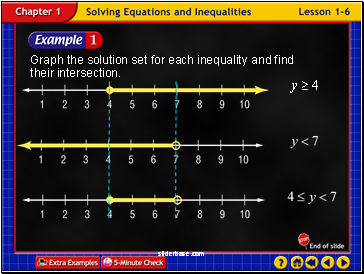Example 6-1b

Graph the solution set for each inequality and find their intersection.

Slide 94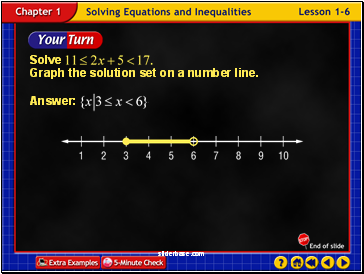Example 6-1c

Slide 95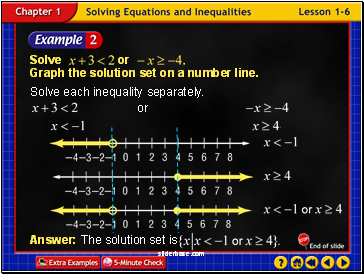Example 6-2a

Solve each inequality separately.

Slide 96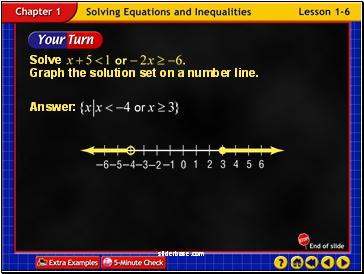Example 6-2b

Slide 97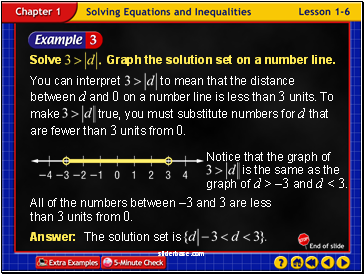Example 6-3a

All of the numbers between –3 and 3 are less than 3 units from 0.

Slide 98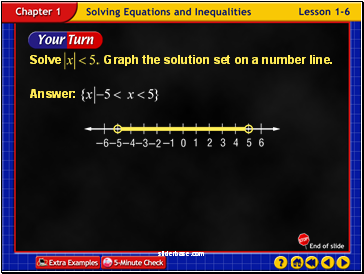Example 6-3b

Slide 99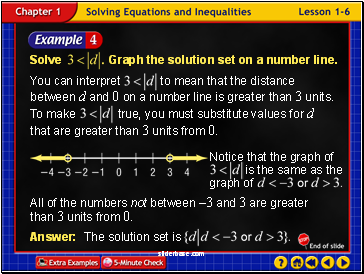Example 6-4a

All of the numbers not between –3 and 3 are greater than 3 units from 0.

Slide 100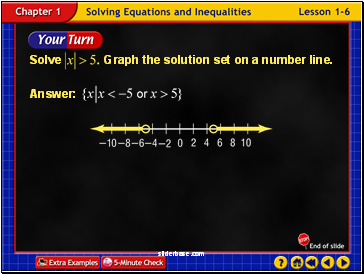Example 6-4b

Slide 101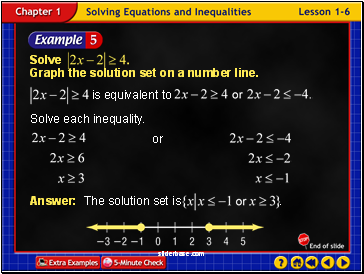Example 6-5a

Solve each inequality.

Slide 102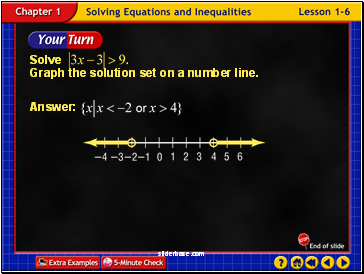Example 6-5b

Slide 103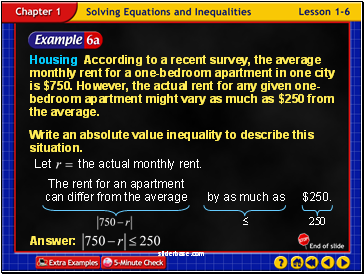Example 6-6a

Housing According to a recent survey, the average monthly rent for a one-bedroom apartment in one city is \$750. However, the actual rent for any given one-bedroom apartment might vary as much as \$250 from the average.

Write an absolute value inequality to describe this situation.

Slide 104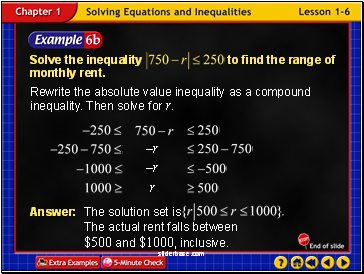Example 6-6b

Rewrite the absolute value inequality as a compound inequality. Then solve for r.

Slide 105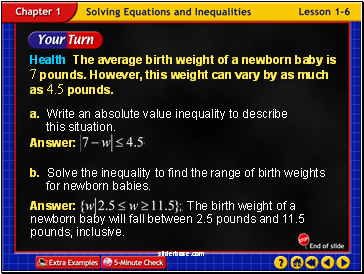Health The average birth weight of a newborn baby is 7 pounds. However, this weight can vary by as much as 4.5 pounds.

a. Write an absolute value inequality to describe this situation.

b. Solve the inequality to find the range of birth weights for newborn babies.

Example 6-6c

Slide 106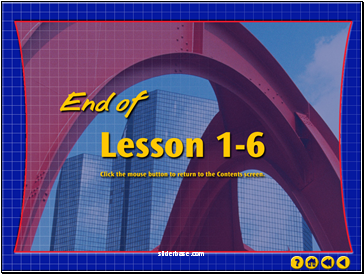End of Lesson 6

Slide 107Algebra2.com

Slide 108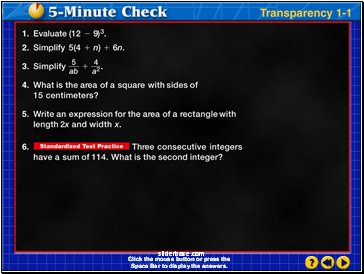Go to page:
1  2  3  4  5  6  7  8